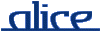# alice library manual.# The Lock structure

## ________ Synopsis ____________________________________________________

```    signature LOCK
structure Lock : LOCK
```

The Lock structure defines a simple means to implement monitor-like synchronisation of arbitrary sets of functions or whole modules.

See the example at the and of this page for a common idiom for building up such synchronisation.

## ________ Import ______________________________________________________

Imported implicitly.

## ________ Interface ___________________________________________________

```    signature LOCK =
sig
type lock
type t = lock

val lock : unit -> lock
val sync : lock -> ('a -> 'b) -> ('a -> 'b)

functor Sync (val lock : lock signature S  structure X : S) : S
end
```

## ________ Description _________________________________________________

type lock
type t = alt

The type of synchronisation locks.

lock ()

Creates a new lock.

sync lock f

Returns a function f', that has the same behaviour as f, but is synchronised with respect to lock. Only one function per lock can be evaluated at one time, other threads will block until that function returns. Locking is not reentrant.

Sync (val lock = lock signature S = S structure X = X)

returns a wrapper of structure X where all fields of function type are synchronised with respect to lock lock. A function that returns a function will return a synchronised function, respectively (i.e. the wrapping is recursively performed for curried functions, such that each partial application is again synchronised).

If X is a functor, then the functor itself is synchronised. When the functor is applied, the resulting module will be wrapped recursively (i.e. the wrapping is recursively performed for curried functors and any resulting structure).

Example:

```      Sync (val lock = lock ()
signature S = (val x : int val f : int -> int val g : int -> int -> int)
structure X = (val x = 5   fun f n = n + 1    fun f n m = n + m))```

returns a structure that is equivalent to

```      struct
val x = 5
val f = sync lock (fn n => n + 1)
val g = sync lock (fn n => sync lock (fn m => n + m))
end```

Similarly,

```      Sync (val lock = lock ()
signature S = fct () -> (val x : int val f : int -> int -> int)
structure X = fct () => (val x = 5   fun f n m = n + m))```

returns a synchronised functor equivalent to

`      fct () => (val x = 5  val f = sync lock (fn n => sync lock (fn m => n + m)))`

Note that structure fields of non-function type will not be wrapped, even if they contain function values. For example,

```      Sync (val lock = lock ()
signature S = (val p : (int -> int) * (int -> int))
structure X = (val p = (fn n => n, fn n => n + 1)))```

returns X unchanged.

## ________ Example _____________________________________________________

The following structure provides two synchronised functions even and odd. Only one thread can run within the implementation of these functions.

```    signature MONITOR =
sig
val even : int -> unit
val odd :  int -> unit
end

structure Monitor : MONITOR =
struct
fun even 0 = ()
| even n = odd(n-1)
and odd 0  = ()
| odd n  = even(n-1)

val lock = Lock.lock()
val even = Lock.sync lock even
val odd  = Lock.sync lock odd
end
```

Alternatively, the structure can be constructed like this:

```    structure Monitor' =
struct
fun even 0 = ()
| even n = odd(n-1)
and odd 0  = ()
| odd n  = even(n-1)
end

structure Monitor = Lock.Sync (val lock = Lock.lock()
signature S = MONITOR
structure X = Monitor')
```